# Closed category

(diff) ← Older revision | Latest revision (diff) | Newer revision → (diff)

A category with an additional structure, thanks to which the internal Hom-functor can be used as a right-adjoint functor to the abstract tensor product.

A categoryis said to be closed if a bifunctor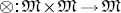(see Functor) and a distinguished objecthave been given on it, and if it admits natural isomorphisms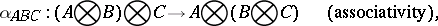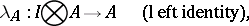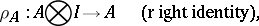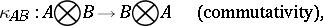such that the following conditions are satisfied: 1) the natural isomorphisms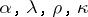are coherent; and 2) every functor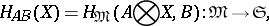where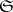is the category of sets, is representable. The representing objects are usually denoted by, and they can be regarded as the values of the bifunctor(the internal Hom-functor) on objects. If the bifunctor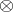coincides with a product andis a right zero (terminal object) of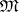, thenis called a Cartesian-closed category.

The following categories are Cartesian closed: the category of sets, the category of small categories and the category of sheaves of sets over a topological space. The following categories are closed: the category of modules over a commutative ring with an identity and the category of real (or complex) Banach spaces and linear mappings with norm not exceeding one.

How to Cite This Entry:
Closed category. Encyclopedia of Mathematics. URL: http://encyclopediaofmath.org/index.php?title=Closed_category&oldid=16972
This article was adapted from an original article by M.Sh. Tsalenko (originator), which appeared in Encyclopedia of Mathematics - ISBN 1402006098. See original article